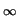Next: D1.3 Failure Criterion Up: D1. Iteration Blocks Previous: D1.1.1 Segment and Device

## D1.2 Termination Criterion

The termination criterion defines under which conditions the block can be considered to be successfully solved. This is normally the case when the update and residuum norms fall under user-specified values.

Block
{
while = updateNorm > finalNorm && rhsNorm > rhsFinalNorm;
}

Another possibility is to terminate a block when a maximum block iteration counter is exceeded as is the case, e.g., for the Gummel scheme  where each iteration block is solved only once.

Block
{
while = iterationCount < 1;
}


The keywords updateNorm and rhsNorm are inherited from the Extern section, while finalNorm and rhsFinalNorm stem from IterateConfig. As updateNorm and rhsNorm contain all quantities of the solution vector their use might be problematic. Although the quantities are scaled to be approximately of the same order the range of possible values is still different. It might therefore be useful for special applications to refine the termination criterion by using only parts of the solution vector. These parts can be accessed through one of the following functions:

• updateNorm2(quantityList) gives the E2 norm of the updates.
• updateNormMax(quantityList) gives the Enorm of the updates.
• rhsNorm2(quantityList) gives the E2 norm of the right-hand-side of the respective part of the equation system.
• rhsNormMax(quantityList) gives the Enorm of the right-hand-side of the respective part of the equation system.
In all cases quantityList is a comma-separated list of quantity class names. Optional arguments of all functions are the name of the block and whether the value should be returned in internal or SI units. E.g., as the potential is scaled to the temperature voltage VT, the value returned is normally a given as multiples of VT while when SI units are requested the values are scaled back with VT. An implementation of Gummel's scheme might look as follows.

DD_Gummel
{
Ele : ~Iterate.Blocks.DDBlock
{
ignoreQuantities = "Potential,*Hole*";
while            = iterationCount < 1;
}
Hol : Ele
{
ignoreQuantities = "Potential,*Electron*";
}
Pot : Ele
{
ignoreQuantities = "*Electron*,*Hole*";
}

while = updateNorm2("*", block = "Ele") +
updateNorm2("*", block = "Hol") +
updateNorm2("*", block = "Pot")   > finalNorm;
}

In the first block (Ele) only the electron continuity equation is solved. This is done by using all models of the DDBlock (keyword models not locally overwritten) but ignoring the potential and all quantity classes matching *Hole* which is true for HoleConcentration and BoundaryHoleCurrent. The latter must be ignored in order to get proper current components as the currents are zero when not solving the continuity equations.

The other blocks solve the hole continuity equation and Poissons equation and for the termination criterion a simple algebraic sum of the block-norms is used.Next: D1.3 Failure Criterion Up: D1. Iteration Blocks Previous: D1.1.1 Segment and Device
Tibor Grasser
1999-05-31